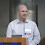## 2012-01-01

### Concrete P-Value Demonstration

I find that students in my statistics class are almost totally bewildered by the logic of hypothesis testing and P-values (for hypotheses based on a population mean), no matter how carefully I try to explain the concepts. Here's an idea for a super-short and simple, concrete demonstration of hypothesis testing. Tell me if you think this would be worth the class time:
1. Start with a hand of four cards: {A, 2, 3, 4}
2. I'll turn my back and secretly do one of two things:
H0: Leave the Ace in, or
HA: Take the Ace out
3. Now shuffle the hand and deal out 3 cards.
Question: Say I get a draw of {2, 3, 4}. What's the chance of this happening if I did not take out the Ace (H0)? Note that all possible draws would be {{A,2,3}, {A,2,4}, {A,3,4}, {2,3,4}} so the probability of seeing that would be P = f/N = 1/4 = 0.25.

Conclusion: If I draw {2,3,4} then we have some evidence that I did change the deck (HA) -- because it's unlikely to see that result if I didn't (P = 0.25).

Now -- You can actually demonstrate this and ask the class if they think I left the Ace in or took it out each time. I'd recommend 3 run-throughs: leave it, leave it, then take it out. (In the latter case, also ask: Is it possible that I left the Ace in?) In reality, you should probably hold the cards against the otherwise full box, so it isn't obvious if your hand becomes empty in the take-it-out case. (And otherwise practice the prestidigitation in advance so your handwork doesn't give it away.)

Open Question: Should I actually reveal to the class which one I did each time (for confirmation), or leave that as a mystery (modeling real-world usage)?

1.I'm struggling with your suggested demonstration, and I think it's because you mention hypothesis testing for means and then proceed with a demonstration that isn't about means. Also, I don't think it's as simple as defining a population then considering all the possible samples. That might make for an effective demonstration, but (as I understand it) hypothesis testing is totally unaware of the size of the population (i.e., your samples of 3 cards do not "know" they're sampling a population of 4 cards). By trying to define all possible samples, I fear students might be misled about the population-sample relationship in hypothesis testing and the theoretical nature of a sampling distribution.

I'm glad you're making me thing about this, because in my limited experience I haven't used much to explain the concept other than drawings of overlapping sampling distributions, and the general explanation that lots of overlap would be higher p-values, and little overlap would be small p-values. I'm guessing there might be some computer simulations that would be helpful, but I haven't explored enough (yet) to find them.

2.Raymond -- Thanks for the comment, really good stuff to think about!

Now, I actually think one of the advantages here is to have an example that is about something other than testing a population mean. One of the things I struggle with in the introductory class is in trying to communicate that the concepts of confidence-intervals and hypothesis-tests apply to a whole universe of parameters other than just a mean (median, standard deviation, proportion, odds ratio, etc.) So dealing with those general concepts in isolation, prior to introducing the machinery of means-testing, I think might give valuable added perspective.

And I think that part of the demonstration is that somehow you do indeed have to categorize all possible sampling results under the null-hypothesis. For this brief example, you can list them individually. For the case of a mean from an unknown population, the analogy is to use the Central Limit Theorem, and conclude that they are at least approximately normally distributed (for a sufficiently large sample). So there is a correspondence there that I'm consciously trying to highlight.